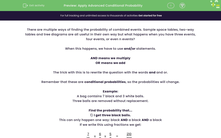### Comprehensive & curriculum aligned

In this worksheet, students will practise finding the probability of multiple conditional probabilities using and/or statements.Key stage:  KS 4

Year:  GCSE

GCSE Subjects:   Maths

GCSE Boards:   AQA, Eduqas, Pearson Edexcel, OCR,

Curriculum topic:   Probability

Curriculum subtopic:   Probability Combined Events and Probability Diagrams

Difficulty level:#### Worksheet Overview

There are multiple ways of finding the probability of combined events. Sample space tables, two-way tables and tree diagrams are all useful in their own way but what happens when you have three events, four events, or even n events?

When this happens, we have to use and/or statements.

AND means we multiply

The trick with this is to rewrite the question with the words and and or.

Remember that these are conditional probabilities, so the probabilities will change.

Example:

A bag contains 7 black and 3 white balls.

Three balls are removed without replacement.

Find the probability that...

1) I get three black balls.

This can only happen one way: black AND a black AND a black

If we write this using fractions we get:

 7 10
x
 6 9
x
 5 8
=
 210 720

This fraction will cancel down to 7/24

2) I get 2 black and 1 white ball.

There are actually three different ways this can happen:

black AND a black AND a white

OR

black AND a white AND a black

OR

white AND a black AND a black

The trick here is to know that each one of these probabilities will actually be the same (try it, you'll see it's true) so we only have to find one.

Let's find black AND a black AND a white

 7 10
x
 6 9
x
 3 8
=
 126 720

We can now say that the probability of getting two blacks and a white is:

 126 720
+
 126 720
+
 126 720
=
 378 720

This will cancel down to 21/40

3) The probability of getting at least one white.

For this, you could work out the probability of each one that satisfies this and add them all together  - there's 7 ways this could happen.

or

You could use the fact that P(at least 1) = 1 - P(none)

So P(at least 1 white) = 1 - P(no whites)

P(at least 1 white) = 1 - P(3 blacks)

We worked out earlier that P(3 blacks) = 7/24

So we can say that the probability of getting at least one white = 1 - 7/24 = 17/24

Let's move on to try some questions now.

### What is EdPlace?

We're your National Curriculum aligned online education content provider helping each child succeed in English, maths and science from year 1 to GCSE. With an EdPlace account you’ll be able to track and measure progress, helping each child achieve their best. We build confidence and attainment by personalising each child’s learning at a level that suits them.

Get started